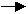CH'AN CULTIVATION -by Professor Yu Kuo K'ung 3. Understanding of Nature and Dharma The self-nature is Ch'an and Ch’an is the self nature. Hence, understanding the characteristics of the word "nature" is essential. Because of the wrong emphasis in our education, we have used end trained our minds mainly to understand and analyze the external world. We have learned a great deal about the physical environment, yet we understand close to nothing about our minds. Therefore, I choose a physical example to illustrate the essential characteristic of nature.       We can run a uniaxial test on a metal bar on a test machine in any mechanics laboratory. However, to make it accessible to everyone, I choose an ordinary rubber band about five inches in diameter. Hang the rubber band and apply increments of load as shown in Fig. 1a. Two points A and B, one inch apart, are marked on the rubber band when the load F=200 grains. This initial load is to reduce the slackness of the rubber band. We define =F - 200 and =x -1. Ten pairs of values of (σ, ε) are carefully measured. These data are plotted and the straight line which is the best to represent the experimental data is obtained by the least square method as shown in Fig. 1b. The experimental curve between O and E can be represented by the following formula: σ= k ε                           (3-1) From the experimental curve, we read that σ = 1,000 gr. (grains) at ε = 0.06 in (inch). Hence, k = 1,000/0.06 = 16,666.67 gr./in. This value of k is called the spring constant of the rubber band. The spring constant is a property or nature of the rubber band. If we choose any other pair of (σ, ε) from the curve, we obtain the same value of the spring constant k. To emphasize this important notion, let us answer the following sequence of questions slowly and attentively: What is the value use of k as σ =1,000 gr.? Answer: k=16,000.67 gr./in. This is what we just calculated. What is the value of k as σ =1,000/2 =500 gr.? Answer: k=16,666.67 gr./in. What is the value of k as σ =1,000/3 =333.33gr.? Answer: k=16,666.67 gr./in. What is the value of k as σ =1,000/N with N∞ ?